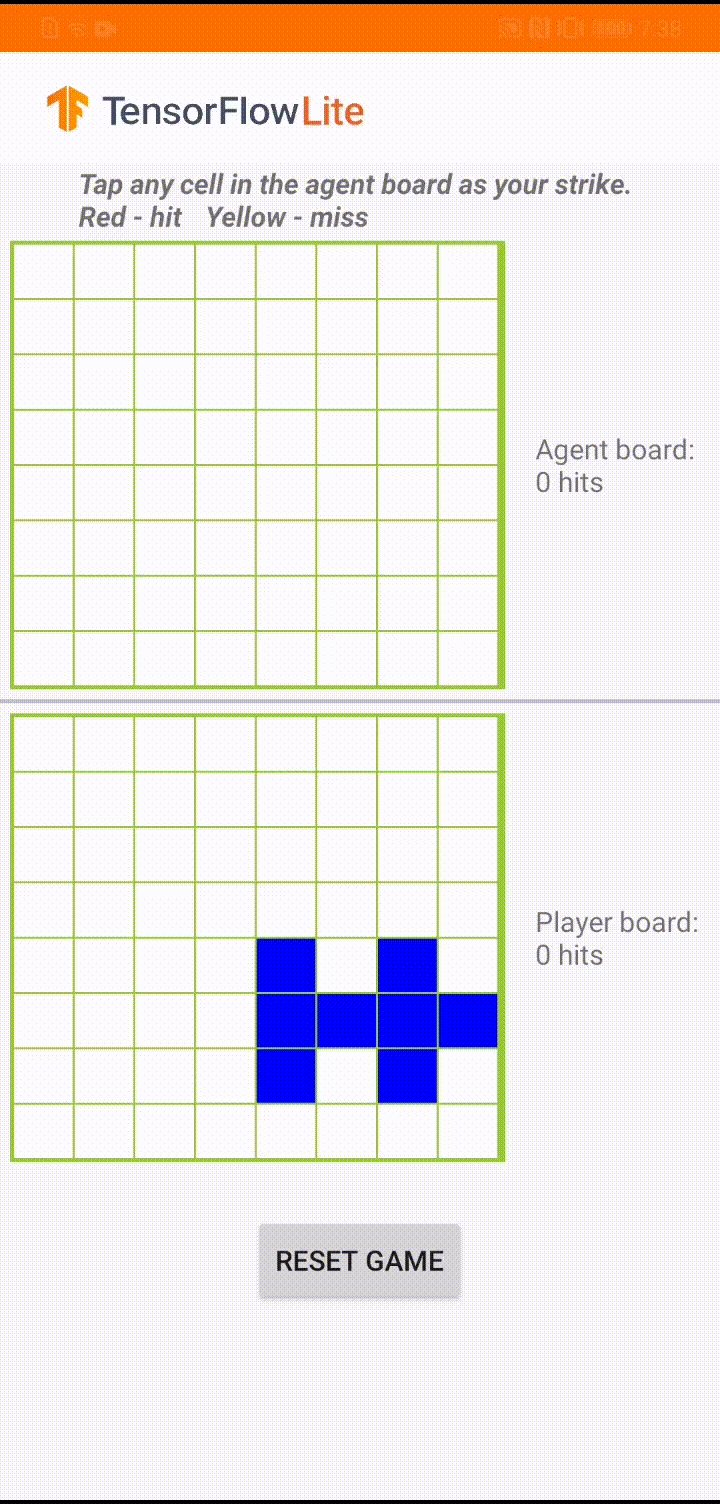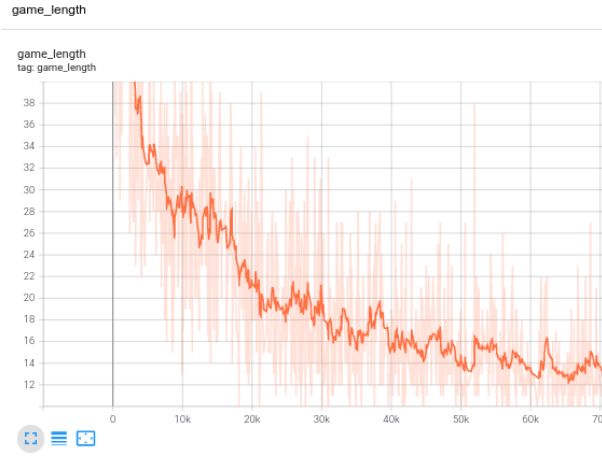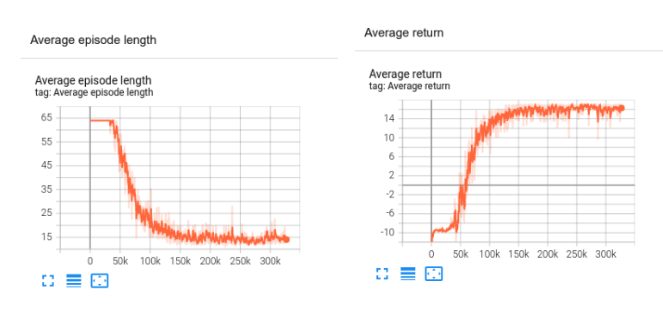一个新的 TensorFlow Lite 示例应用：棋盘游戏Plane Strike 游戏演示

• 与在游戏开始时，用户和 agent 在自己的棋盘上各有一个“飞机”对象（如上方动图所示，8 个蓝色单元格组成“飞机”）；这些飞机只对棋盘所有者可见，而对其对手是隐藏的。

• 用户和 agent 轮番攻击对方棋盘，每次攻击一个单元格。用户可以点击 agent 棋盘上的任何单元格，而 agent 将根据机器学习模型的预测自动做出选择。如果点击的单元格是组成“飞机”的单元格（“命中”），则单元格会变为红色；否则将会变成黄色（“未命中”）。

• 首先击中 8 个红色单元格的一方赢得比赛；然后刷新棋盘，重新开始游戏。

从零开始构建模型• T ：每段的时步数，各段的时步数可能有所不同
• st ：时步上的状态 t
• at ：时步上的所选操作 t 指定状态 s
• πθ ：参数为 θ 的策略
• R(*) ：在指定策略下，收集到的奖励

model.compile(loss='sparse_categorical_crossentropy', optimizer=sgd)

model.fit(x=board_log, y=action_log, sample_weight=rewards)TensorBoard 中的训练进度

converter = tf.lite.TFLiteConverter.from_keras_model(model)
tflite_model = converter.convert()

convertBoardStateToByteBuffer(board);
tflite.run(boardData, outputProbArrays);
float[] probArray = outputProbArrays;
int agentStrikePosition = -1;
float maxProb = 0;
for (int i = 0; i < probArray.length; i++) {
int x = i / Constants.BOARD_SIZE;
int y = i % Constants.BOARD_SIZE;
if (board[x][y] == BoardCellStatus.UNTRIED && probArray[i] > maxProb) {
agentStrikePosition = i;
maxProb = probArray[i];
}
}

使用 TensorFlow Agents 训练的模型

actor_net = tfa.networks.Sequential([
tfa.keras_layers.InnerReshape([BOARD_SIZE, BOARD_SIZE], [BOARD_SIZE**2]),
tf.keras.layers.Dense(FC_LAYER_PARAMS, activation='relu'),
tf.keras.layers.Dense(BOARD_SIZE**2),
tf.keras.layers.Lambda(lambda t: tfp.distributions.Categorical(logits=t)),
],        input_spec=train_py_env.observation_spec(
))

tf_agent = reinforce_agent.ReinforceAgent(
train_env.time_step_spec(),
train_env.action_spec(),
actor_network=actor_net,
optimizer=optimizer,
normalize_returns=True,
train_step_counter=train_step_counter)

def collect_episode(environment, policy, num_episodes, replay_buffer_observer):
"""Collect game episode trajectories."""
initial_time_step = environment.reset()

driver = py_driver.PyDriver(
environment,
py_tf_eager_policy.PyTFEagerPolicy(policy, use_tf_function=True),
[replay_buffer_observer],
max_episodes=num_episodes)
initial_time_step = environment.reset()
driver.run(initial_time_step)

for i in range(iterations):
# Collect a few episodes using collect_policy and save to the replay buffer.
collect_episode(train_py_env, collect_policy,
COLLECT_EPISODES_PER_ITERATION, replay_buffer_observer)

# Use data from the buffer and update the agent's network.
iterator = iter(replay_buffer.as_dataset(sample_batch_size=1))
trajectories, _ = next(iterator)
tf_agent.train(experience=trajectories)
replay_buffer.clear()TensorBoard 中 TF agent 的训练进度

converter = tf.lite.TFLiteConverter.from_saved_model(
policy_dir, signature_keys=['action'])
converter.target_spec.supported_ops = [
tf.lite.OpsSet.TFLITE_BUILTINS,  # enable TensorFlow Lite ops.
tf.lite.OpsSet.SELECT_TF_OPS  # enable TensorFlow ops.
]
tflite_policy = converter.convert()
with open(os.path.join(model_dir, 'planestrike_tf_agents.tflite'), 'wb') as f:
f.write(tflite_policy)

@Override
protected void runInference() {
Map output = new HashMap<>();
// TF Agent directly returns the predicted action
int[][] prediction = new int;
output.put(0, prediction);
tflite.runForMultipleInputsOutputs(inputs, output);
agentStrikePosition = prediction;